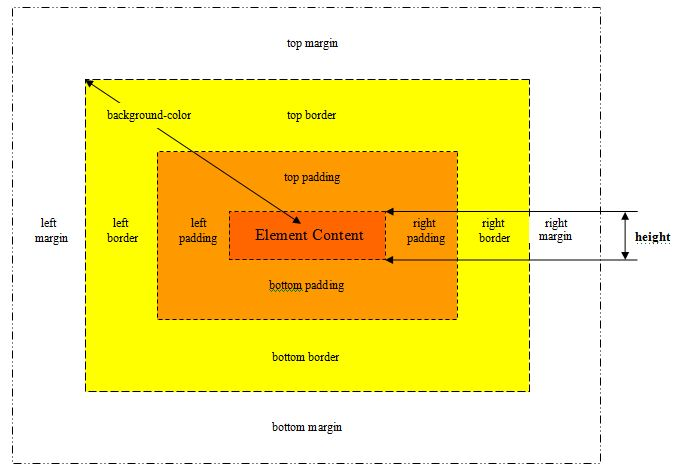# `.height()` JQ Home  <<  A & P  <<  .height()

First element height retrieval and matched set height setting.

### Description

The `.height()` method is used to retrieve the currently computed CSS content height of the first element within the matched or set the CSS content height of every matched element.

• Useful in mathematical calculations as any unit measurements are stripped off.
• The height only relates to the element content and does not include any padding, border or margin.### Syntax

Signature Description
`.height() `Retrieve the currently computed CSS height from the first element within the matched set.
`.height( value ) `Set the CSS height of each element within the matched set.
`.height( function( index, value ) )`A function returning the CSS height to set.

### Parameters

Parameter Description Type
`value`Either an integer representing the new height in pixels or a string containing the new height as an integer with a CSS recognized unit measurement appended to it.`String` or
`Number`
`function(index, value)`A function.
• Each time the callback runs, it is passed the current index position of the element in the set.
• The existing height pertaining to the current element is also passed.
• The callback is fired in the context of the current element in the set, so the keyword `this` refers to that element.
`Function`

### Return

Retrieving - A `Number` object (an integer).

Setting - A `jQuery` object including the updated height within the matched set.

### `.height()` Example A & P  <<  Top

Retrieve the currently computed CSS content height from the first element within the matched set.

• The `.height()` signature will always return the content height, regardless of the value of the CSS `box-sizing property`.

In the example below when we press the button we get the heights of the table and the table row below.

 Table Row 1, Table Data 1 Table Row 1, Table Data 2

``````
\$(function(){
\$('#btn1').on('click', function() {
alert('The table has a height of ' +  \$('#table1').height()
+ ' and the table row has a height of ' + \$('#table1 tr').height());
});
});

``````

Press the button below to action the above code:

### `.height( value )` Example A & P  <<  Top

Set the CSS height of each element within the matched set.

• The `.height( value )` signature sets the height of the box in accordance with the `CSS box-sizing property` and if this is set to `border-box` it will cause this function to change the outerHeight of the box instead of the content height.

In the example below when we press the left button we make the table rows bigger.

When we press the left button we make the table rows smaller.

 Table Row 1, Table Data 1 Table Row 1, Table Data 2

``````
\$(function(){
\$('#btn2').on('click', function() {
\$('#table2 tr').height(100);
});
\$('#btn3').on('click', function() {
\$('#table2 tr').height(50);
});
});

``````

Press the button below to action the above code:

### `.height( function(index, value) )` Example A & P  <<  Top

A function returning the CSS height to set.

In the example below when the button is pressed we return a new height for index 1 (table row 2).

 Table Row 1, Table Data 1 Table Row 1, Table Data 2 Table Row 2, Table Data 1 Table Row 2, Table Data 2 Table Row 3, Table Data 1 Table Row 3, Table Data 2

``````
\$(function(){
\$('#btn4').on('click', function() {
\$('#table3 tr').height( function(index, value) {
if (index == 1) {
return \$(this).height(100);
}
});
});
});

``````

Press the button below to action the above code:

### Related Tutorials

jQuery Basic Tutorials - Lesson 11 - Working With Dimension & Position CSS Properties